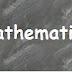## IIT JEE Mathematics Free Study Material 2017

Mathematics free study material for IIT-JEE 2017 in PDF eBook format is very easy to read and help the readers to understand the basics. It is very important material for those student who are preparing for IIT-JEE Mains & Advance and other state level Examinations.

In this study material we have provided theory as well as solved examples by which an average student can also grasp the basic concepts described it the material after that some exercises are also given at the last of the each topic.

But I will advise you that first understand the basics concepts and then try to solve NCERT to check your Level after that you can switch to Various Famous books like Objective Mathematics by RD Sharma and Objective Mathematics by S.K. Goyal.

Mathematics study material download links are given in part wise manner.

Functions

Probability

Trigonometry ratio & Identities

Properties of Triangles

Point and Straight line

Circle

Conic Sections

Vector & 3D

Sequence & Series

Permutation & Combinations

Binomial Theorem

Complex Number

Limits

Continuity

Differentiation

Application of Derivatives

Integration

Area under Curve

Differential Equation

IIT JEE Mathematics Study Material Free pdf ebooks downloadReviewed by Unknown on 07:16 Rating: 5